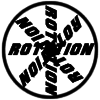#### You may also like### Reflect Again

Follow hints to investigate the matrix which gives a reflection of the plane in the line y=tanx. Show that the combination of two reflections in intersecting lines is a rotation.### Rots and Refs

Follow hints using a little coordinate geometry, plane geometry and trig to see how matrices are used to work on transformations of the plane.### Sine Problem

In this 'mesh' of sine graphs, one of the graphs is the graph of the sine function. Find the equations of the other graphs to reproduce the pattern.

# Squaring the Rectangle

##### Age 14 to 18 Challenge Level:
Draw a rectangle on a piece of paper, and cut it out.

Can you find a way to cut your rectangle into pieces that can be reassembled to make a square?

Try to do it using the smallest number of pieces you can.

Is it always possible to cut up and reassemble a rectangle into a square using finitely many pieces?

Start with a rectangle with dimensions in the ratio 2:1. What side length do you need your square to have? How could you construct it?

Start with a square. How many different ways can you find to cut it into two pieces to make a rectangle? Or three pieces?

Click below to find an interactivity which shows one way of cutting up and reassembling a square to make a rectangle.

Can you move the pieces to make a square?
Can you move the pieces to make a rectangle?

Slide the point on the side of the square to change the pieces.
Move and rotate each piece using the blue dots.

Can you work out how the pieces were constructed?